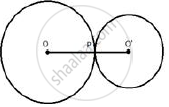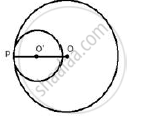Share

Books Shortlist

# Radii of Two Circles Are 6. 3 Cm and 3.6 Cm. State the Distance Between Their Centres If: (I) They Touch Each Other Externally (Ii) They Touch Each Other Internally - ICSE Class 10 - Mathematics

ConceptTangent Properties - If Two Circles Touch, the Point of Contact Lies on the Straight Line Joining Their Centers

#### Question

Radii of two circles are 6. 3 cm and 3.6 cm. State the distance between their centres if:
(i) they touch each other externally
(ii) they touch each other internally

#### Solution

Radius of bigger circle = 6.3 cm
and of smaller circle = 3.6 cm

i)Two circles are touching each other at P externally. O and O ' are the centers of the circles. Join
OP and O'P
OP = 6.3 cm, O'P = 3.6 cm
OP + O'P = 6.3 + 3.6 = 9.9 cm

ii)Two circles are touching each other at P internally. O and O ' are the centers of the circles. Join
OP and O’P
OP = 6.3 cm, O'P = 3.6 cm
OO ' = OP − O’P = 6.3 − 3.6 = 2.7 cm

Is there an error in this question or solution?

#### APPEARS IN

Solution Radii of Two Circles Are 6. 3 Cm and 3.6 Cm. State the Distance Between Their Centres If: (I) They Touch Each Other Externally (Ii) They Touch Each Other Internally Concept: Tangent Properties - If Two Circles Touch, the Point of Contact Lies on the Straight Line Joining Their Centers.
S